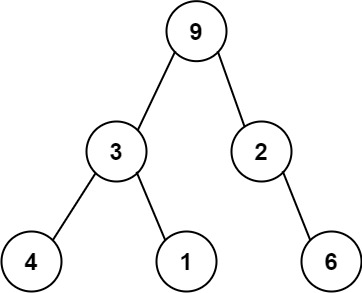## Algorithm

Problem Name: 331. Verify Preorder Serialization of a Binary Tree

One way to serialize a binary tree is to use preorder traversal. When we encounter a non-null node, we record the node's value. If it is a null node, we record using a sentinel value such as `'#'`.For example, the above binary tree can be serialized to the string `"9,3,4,#,#,1,#,#,2,#,6,#,#"`, where `'#'` represents a null node.

Given a string of comma-separated values `preorder`, return `true` if it is a correct preorder traversal serialization of a binary tree.

It is guaranteed that each comma-separated value in the string must be either an integer or a character `'#'` representing null pointer.

You may assume that the input format is always valid.

• For example, it could never contain two consecutive commas, such as `"1,,3"`.

Note: You are not allowed to reconstruct the tree.

Example 1:

```Input: preorder = "9,3,4,#,#,1,#,#,2,#,6,#,#"
Output: true
```

Example 2:

```Input: preorder = "1,#"
Output: false
```

Example 3:

```Input: preorder = "9,#,#,1"
Output: false
```

Constraints:

• `1 <= preorder.length <= 104`
• `preorder` consist of integers in the range `[0, 100]` and `'#'` separated by commas `','`.

## Code Examples

### #1 Code Example with C++ Programming

```Code - C++ Programming```

``````
class Solution {
public:
bool isValidSerialization(string preorder) {
if(preorder == "#") return true;
stringstream ss(preorder);
stackstk;
string s = "";
while(getline(ss, s, ',')){
if(s == "#" && stk.empty()) return false;
if(!stk.empty()) stk.top()++;
if(!stk.empty() && stk.top() == 2) stk.pop();
if(s != "#") stk.push(0);
if(stk.empty() && !ss.eof()) return false;
}
return stk.empty();
}
};
``````
Copy The Code &

Input

cmd
preorder = "9,3,4,#,#,1,#,#,2,#,6,#,#"

Output

cmd
true

### #2 Code Example with Java Programming

```Code - Java Programming```

``````
class Solution {
public boolean isValidSerialization(String preorder) {
String[] nodes = preorder.split(",");
int diff = 1;
for (String node : nodes) {
if (--diff < 0) {
return false;
}
if (!node.equals("#")) {
diff += 2;
}
}
return diff == 0;
}
}
``````
Copy The Code &

Input

cmd
preorder = "9,3,4,#,#,1,#,#,2,#,6,#,#"

Output

cmd
true

### #3 Code Example with Javascript Programming

```Code - Javascript Programming```

``````
const isValidSerialization = function(preorder) {
const nodes = preorder.split(',')
let diff = 1
for(let node of nodes) {
if(--diff < 0) return false
if(node !== '#') diff += 2
}
return diff === 0
};
``````
Copy The Code &

Input

cmd
preorder = "1,#"

Output

cmd
false

### #4 Code Example with Python Programming

```Code - Python Programming```

``````
class Solution:
def isValidSerialization(self, preorder: str) -> bool:
stack = []
for c in preorder.split(','):
stack.append(c)
while stack[-2:] == ['#', '#']:
stack.pop()
stack.pop()
if not stack: return False
stack.pop()
stack.append('#')
return stack == ['#']
``````
Copy The Code &

Input

cmd
preorder = "1,#"

Output

cmd
false